NEET  >  Pressure-Volume Work: Reversible & Irreversible Isothermal

# Pressure-Volume Work: Reversible & Irreversible Isothermal - Notes | Study Chemistry Class 11 - NEET

 1 Crore+ students have signed up on EduRev. Have you?

EXPRESSION FOR PRESSURE – VOLUME WORK
1. Work Done in Reversible Isothermal Expansion: Consider an ideal gas enclosed in a cylinder fitted with a weightless and frictionless piston. The cylinder is not insulated.
The external pressure, Pext, is equal to pressure of the gas, Pgas Let it be P.
Pext = Pgas = P
If the external pressure is decreased by an infinitesimal amount dP, the gas will expand by an infinitesimal volume, dV.

As a result of expansion, the pressure of the gas within the cylinder falls to Pgas - dP, i.e., it becomes again equal to the external pressure and, thus, the piston comes to rest.

Such a process is repeated for a number of times, i.e., in each step the gas expands by a volume dV.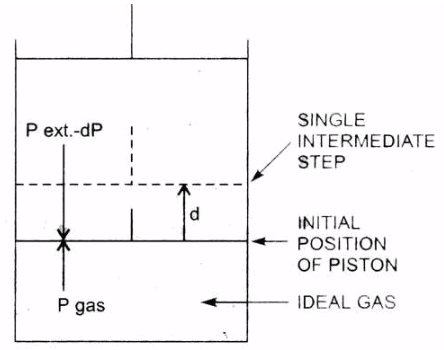Isothernal reversible expansion work

Since, the system is in thermal equilibrium with the surroundings, the infinitesimally small cooling produced due to expansion is balanced by the absorption of heat from the surroundings and the temperature remains constant throughout the expansion.The work done by the gas in each step of expansion can be given as,
dw = -(Pext - dP)dV
= -Pext.dV
= -PdV
dP. dV, the product of two infinitesimal quantities, is neglected.

• The negative sign of this expression is required to obtain conventional sign for w, which will be positive. It indicates that in case of compression work is done on the system.
• Here (V– Vi ) will be negative and negative multiplied by negative will be positive. Hence the sign obtained for the work will be positive.

If the pressure is not constant at every stage of compression, but changes in number of finite steps, work done on the gas will be summed over all the steps and will be equal to -Σp∆V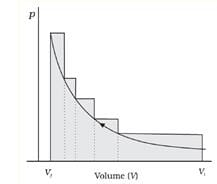pV-plot when pressure is not constant and changes in finite steps during compression from initial volume, Vi to final volume, Vf . Work done on the gas is represented by the shaded area.

• The total amount of work done by the isothermal reversible expansion of the ideal· gas from volume V1 to volume V2 is, therefore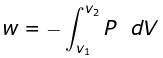For an ideal gas, p = nRT/V
So,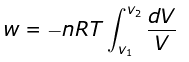Integrating,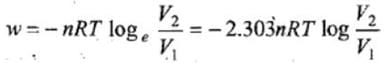At constant temperature, according to Boyle's law,
P1V1 = P2V2
or V2/V1 = P1/P2
So,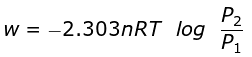Isothermal compression work of an ideal gas may be derived similarly and it has exactly the same value with positive sign.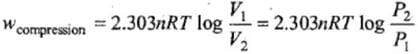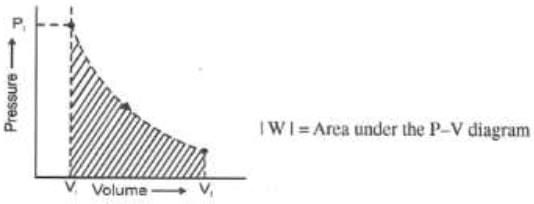WORK DONE IN IRREVERSIBLE ISOTHERMAL EXPANSION

Two types of irreversible isothermal expansions are observed, i.e.,
(i) Free expansion and
(ii) Intermediate expansion.

• In free expansion, the external pressure is zero, i.e. , work done is zero when gas expands in vacuum.
• In intermediate expansion, the external pressure is less than gas pressure. So, the work done when volume changes from V1 to V2 is given by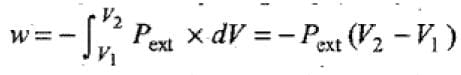Since, Pext is less than the pressure of the gas, the work done during intermediate expansion is numerically less than the work done during reversible isothermal expansion in which Pext is almost equal to Pgas .

ISOTHERMAL AND FREE EXPANSION OF AN IDEAL GAS

For isothermal (T = constant) expansion of an ideal gas into vacuum ; w = 0 since pext = 0.

Also, Joule determined experimentally that q = 0; therefore, ∆U = 0
∆U = q + w can be expressed for isothermal irreversible and reversible changes as follows:
1. For isothermal irreversible change
q = – w = Pext (Vf – Vi )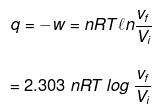3. For adiabatic change, q = 0,

REVERSIBLE ISOTHERMAL COMPRESSION OF AN IDEAL GAS

This can be achieved by placing particles of sand one by one at a very slow take in the assembly which keeps the temperature of gas constant in this case the expression of work done will be exactly similar to as obtained in case of reversible expansion of gas
W= -nRT ln (Vf /Vi)
This will automatically come out to be +ve as Vf < Vi

Example 1. 10 gm of Helium at 127°C is expanded isothermally from 100 atm to 1 atm Calculate the work done when the expansion is carried out
(i) In single step
(ii) In three steps the intermediate pressure being 60 and 30 atm respectively and
(iii) Reversibly.

Solution.
(i) Work done = V.ΔP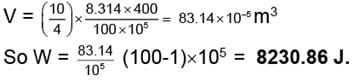(ii) In three steps
VI = 83.14 × 10-5 m3
WI = (83.14 × 10-5) × (100 - 60) × 105
= 3325.6 Jules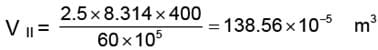WII = V. DP
WII = 138.56 × 10-5 (60 - 30) × 105
= 4156.99 ≈ 4157 J.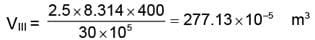WIII = 277.13 × 10-5 (30 - 1) × 105
WIII = 8036.86 J.
W total  =  WI + WII + WIII
= 3325.6 + 4156.909 + 8036.86 = 15519.45 J.
(iii) For reversible process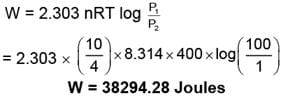Example 2. Calculate the amount of work done by 2 mole of an ideal gas at 298 K in reversible isothermal expansion from 10 litre to 20 litre.

Solution. Amount of work, done in reversible isothermal expansion,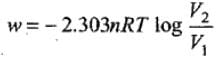Given, n = 2, R = 8.314 JK-1 mol-1, T = 298 K, V2 = 20 L and V1 = 10 L.
Substituting the values in above equation,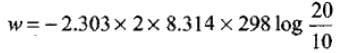= -2.303 × 8.314 × 298 × 0.3010 = -3434.9 J
i.e., work is done by the system.

Example 3. 5 moles of an ideal gas expand isothermally and reversibly film a pressure of 10 atm to 2atm at 300K. What is the largest mass which can be lifted through a height of l metre in this expansion?

Solution. Work done by the system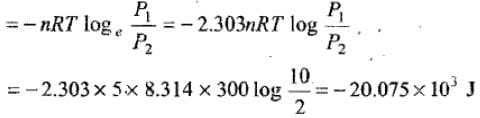Let M be the mas which can be lifted through a height of 1 m.

Work done in lifting the mass = Mgh = M × 9.8 × 11
So, M × 9.8 = 20.075 × 103 M
= 2048.469 kg

The document Pressure-Volume Work: Reversible & Irreversible Isothermal - Notes | Study Chemistry Class 11 - NEET is a part of the NEET Course Chemistry Class 11.
All you need of NEET at this link: NEET

## Chemistry Class 11

204 videos|331 docs|229 tests

## Chemistry Class 11

204 videos|331 docs|229 tests

### How to Prepare for NEET

Read our guide to prepare for NEET which is created by Toppers & the best Teachers

Track your progress, build streaks, highlight & save important lessons and more!

,

,

,

,

,

,

,

,

,

,

,

,

,

,

,

,

,

,

,

,

,

;